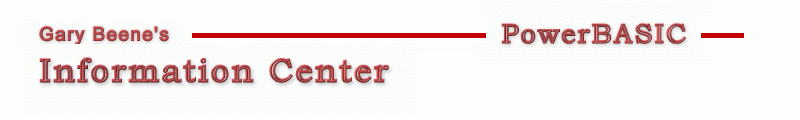## Convert To GrayScale

Category: Bitmaps

Date: 03-28-2012

'While not an everyday need for most programmers, it is sometimes useful
'to convert an image to grayscale. The Graphic Get/Set Pixel statements
'are the simplest approach. But the use of Graphic Get/Set Bits is much faster.

'For visible images, the Window GetPixel and SetPixel API can also be used,
'but are similar in speed to the Graphic Set/Get Pixel statements.

'Primary Code:
'All of the approaches use the same 4 basic steps:
'get Long color value:
'extract color components
'compute gray component
'return grayscale Long value to display

'Graphic Get/Set Pixels:
'get Long color value:
p = StrPTR(bmp\$) + 8 + (y*w+x)*4
iColor = @p
'extract color components
R = iColor Mod 256 : G = (iColor\256) Mod 256 : B = (iColor\256\256) Mod 256
'compute gray component
iColor = 0.299*R + 0.587*G + 0.114*B
'return grayscale Long value to display
@p = iColor

'Graphic Get/Set Bits (Long pointer):
'get Long color value:
Graphic Get Pixel (x,y) To iColor
'extract color components
R = iColor Mod 256 : G = (iColor\256) Mod 256 : B = (iColor\256\256) Mod 256
'compute gray component
iColor = 0.299*R + 0.587*G + 0.114*B
'return grayscale Long value to display
Graphic Set Pixel (x,y), iColor

'GetPixel/SetPixel API:
'get Long color value:
iColor = GetPixel(hDC,x,y)
'extract color components
R = iColor Mod 256 : G = (iColor\256) Mod 256 : B = (iColor\256\256) Mod 256
'compute gray component
iColor = 0.299*R + 0.587*G + 0.114*B
'return grayscale Long value to display
SetPixel(hWnd,x,y,iColor)

'Compilable Example:
'Each of the 3 methods above are demonstrated in this example. Also a variation
'on Graphic Get/Set Bits is shown which uses BYTE instead of Long pointers
#Compiler PBWin 9, PBWin 10
#Compile EXE
#Dim All
#Include "Win32API.inc"
#Resource "gbsnippets.pbr"
Global hDlg As DWord, hBMP As DWord
%ID_Graphic1 = 500
%ID_Graphic2 = 501

Function PBMain() As Long
Dialog New Pixels, 0, "Graphic Get/Set Bits Test",600,300,180,350, %WS_SysMenu, 0 To hDlg
Control Add Button, hDlg, 100, "Convert - Graphic Set", 10,10,150,20
Control Add Button, hDlg, 200, "Convert - SetPixel API", 10,40,150,20
Control Add Button, hDlg, 300, "Convert - Bit String (Long)", 10,70,150,20
Control Add Button, hDlg, 400, "Convert - Bit String (BYTE)", 10,100,150,20
Control Add Button, hDlg, 600, "Reset", 115,130,60,20
Control Add Button, hDlg, 800, "Reset", 115,170,60,20
Control Add Graphic, hDlg, %ID_Graphic1,"", 10,130,100,100, %WS_Visible ' Or %SS_Sunken
Control Add Graphic, hDlg, %ID_Graphic2,"", 10,240,100,100, %WS_Visible 'Or %SS_Sunken
Graphic Attach hDlg, %ID_Graphic1 : Graphic Color %Black,%White : Graphic Clear
Graphic Render "cowgirl", (0,0)-(100,100)
Dialog Show Modal hDlg Call DlgProc
End Function

CallBack Function DlgProc() As Long
If CB.Msg = %WM_Command AND CB.Ctl = 100 Then GrayScale1
If CB.Msg = %WM_Command AND CB.Ctl = 200 Then GrayScale2
If CB.Msg = %WM_Command AND CB.Ctl = 300 Then GrayScale3
If CB.Msg = %WM_Command AND CB.Ctl = 400 Then GrayScale4
If CB.Msg = %WM_Command AND CB.Ctl = 800 Then
Local hbmp as DWord
Control Handle hdlg, %ID_Graphic1 to hbmp
bmp_grayscale hbmp
End If
If CB.Msg = %WM_Command AND CB.Ctl = 600 Then
Graphic Attach hDlg, %ID_Graphic2
Graphic Clear
End If
End Function

Sub GrayScale1   'Graphic Get/Set Pixel statements
Local i,j,R,G,B,w,h,iColor as Long
Graphic Attach hDlg, %ID_Graphic1
Graphic Get Scale To i,j,w,h
For i = 0 to w-1
For j = 0 to h-1
Graphic Attach hDlg, %ID_Graphic1
Graphic Get Pixel (i,j) To iColor  'RGB byte positions are 0-B-G-R
R = iColor Mod 256            'or  iColor AND &HFF&
G = (iColor\256) Mod 256      'or  (iColor AND &HFF00&) \ &H100
B = (iColor\256\256) Mod 256  'or  (iColor AND &HFF0000&) \ &H10000&
iColor = 0.299*R + 0.587*G + 0.114*B  'or this: iColor = (R+G+B)/3
Graphic Attach hDlg, %ID_Graphic2
Graphic Set Pixel (i,j), Rgb(iColor,iColor,iColor)
Next j
Next i
End Sub

Sub GrayScale2   'GetPixel and SetPixel API
Local i,j,R,G,B,w,h,iColor as Long
Local hDC1, hDC2 as DWord
Graphic Attach hDlg, %ID_Graphic1
Graphic Get Scale To i,j,w,h
Graphic Get DC To hDC1
Graphic Attach hDlg, %ID_Graphic2
Graphic Get DC To hDC2
For i = 0 to w-1
For j = 0 to h-1
iColor = GetPixel(hDC1,i,j)   'RGB byte positions are 0-B-G-R
R = iColor Mod 256            'or  iColor AND &HFF&
G = (iColor\256) Mod 256      'or  (iColor AND &HFF00&) \ &H100
B = (iColor\256\256) Mod 256  'or  (iColor AND &HFF0000&) \ &H10000&
iColor = 0.299*R + 0.587*G + 0.114*B  'or this: iColor = (R+G+B)/3
SetPixel(hDC2,i,j,Rgb(iColor,iColor,iColor))
Next j
Next i
Graphic Redraw
End Sub

Sub GrayScale3   'uses Long pointer/CVL solution
Local w As Long, h As Long, p As Long Ptr, i As Long
Local iColor As Long, R As Long, G As Long, B As Long, bmp\$

'get the string from ID_Graphic1
Graphic Attach hDlg, %ID_Graphic1
Graphic Get Bits To bmp\$

'get width/height of image
w = CVL(bmp\$,1)
h = CVL(bmp\$,5)
p = StrPTR(bmp\$)+8    'position of starting position for bits in string

'get string position of coordinates and modify the string at that position
For i = 1 to w*h
iColor = @p                           'result is a BGR color value 0-R-G-B
B = iColor Mod 256                    'or this: iColor AND &HFF&
G = (iColor\256) Mod 256              'or this: (iColor AND &HFF00&) \ &H100
R = (iColor\256\256) Mod 256          'or this: (iColor AND &HFF0000&) \ &H10000&
iColor = 0.299*R + 0.587*G + 0.114*B  'or this: iColor = (R+G+B)/3
@p = Bgr(iColor, iColor, iColor)           'modify string at that position
Incr p
Next i

'put the modified string into ID_Graphic2
Graphic Attach hDlg, %ID_Graphic2
Graphic Set Bits bmp\$
End Sub

Sub GrayScale4   'uses BYTE pointer/CVL solution
Local w As Long, h As Long, bp As Byte Ptr, i As Long, p As Long PTR
Local iColor As Long, R As Long, G As Long, B As Long, bmp\$

'get the string from ID_Graphic1
Graphic Attach hDlg, %ID_Graphic1
Graphic Get Bits To bmp\$

'get width/height of image
w = CVL(bmp\$,1)
h = CVL(bmp\$,5)
bp = StrPTR(bmp\$)+8
p = bp

'get string position of coordinates and modify the string at that position
For i = 1 to w*h
B = @bp                      'string BGR bytes positions are 0-R-G-B
Incr bp : G = @bp
Incr bp : R = @bp
Incr bp : Incr bp
iColor = 0.299*R + 0.587*G + 0.114*B  'create gray component
@p = Bgr(iColor,iColor,iColor)        'modify string at that position
Incr p
Next i

'put the modified string into ID_Graphic2
Graphic Attach hDlg, %ID_Graphic2
Graphic Set Bits bmp\$
End Sub

'gbs_00439
'Date: 03-10-2012

Sub BMP_GrayScale (     ByVal hBMP1 As DWord, _
Opt ByVal hBMP2 As DWord  )

Dim G  As Local Long
Dim P  As Local Byte Ptr
Dim S  As Local String
Dim X  As Local Long
Dim XX As Local Long
Dim Y  As Local Long
Dim YY As Local Long

Graphic Attach hBMP1, 0
Graphic Get Client To XX, YY
Graphic Get Bits To S
P = StrPTR(S) + 8
If hBMP2 <> 0 Then
Graphic Attach hBMP2, 0
End If

Decr YY
Decr XX
For Y = 0 To YY
For X = 0 To XX
G =     (@P * 0.299) : Incr P ' Red
G = G + (@P * 0.587) : Incr P ' Green
G = G + (@P * 0.114) : Incr P ' Blue
Incr P
Graphic Set Pixel (X,Y), Rgb(G,G,G)
Next
Next

End Sub

created by gbSnippets
http://www.garybeene.com/sw/gbsnippets.htm